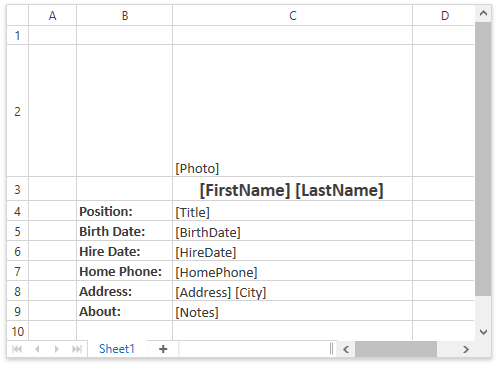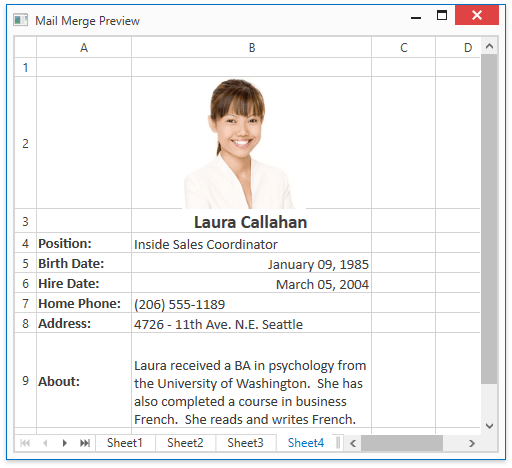# How to: Perform a Mail Merge

This tutorial shows how to use the Spreadsheet Mail Merge functionality to automatically generate a document based on data retrieved from a data source.

1. Create a mail merge template and specify template ranges.

``````// Create a mail merge template.
Worksheet template = workbook.Worksheets;
template.Cells["C2"].Formula = "FIELDPICTURE(\"Photo\", \"range\", C2, FALSE, 50)";
template.Cells["C3"].Formula = "=FIELD(\"FirstName\")&\" \"&FIELD(\"LastName\")";
template.Cells["B4"].Value = "Position:";
template.Cells["C4"].Formula = "FIELD(\"Title\")";
template.Cells["B5"].Value = "Birth Date:";
template.Cells["C5"].Formula = "FIELD(\"BirthDate\")";
template.Cells["C5"].NumberFormat = "MMMM dd, yyyy";
template.Cells["B6"].Value = "Hire Date:";
template.Cells["C6"].Formula = "FIELD(\"HireDate\")";
template.Cells["C6"].NumberFormat = "MMMM dd, yyyy";
template.Cells["B7"].Value = "Home Phone:";
template.Cells["C7"].Formula = "FIELD(\"HomePhone\")";
template.Cells["C9"].Formula = "FIELD(\"Notes\")";

// Set a detail range in the template.
CellRange detail = template.Range["C1:C9"];
detail.Name = "DETAILRANGE";

// Set a header range in the template.
``````

The resulting template is shown in the following image:2. Define the mail merge mode and document orientation. In this example, the mail merge mode is set to Multiple Sheets so that each data record is merged into a separate worksheet in a single workbook.

``````// Set the mail merge mode to "Multiple Sheets".

// Set the document orientation.
``````workbook.MailMergeDataSource = EmployeesInfo.GetData();